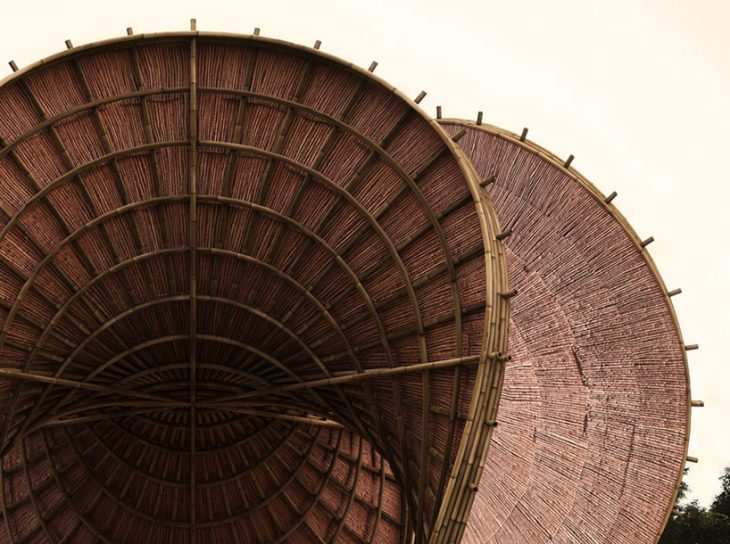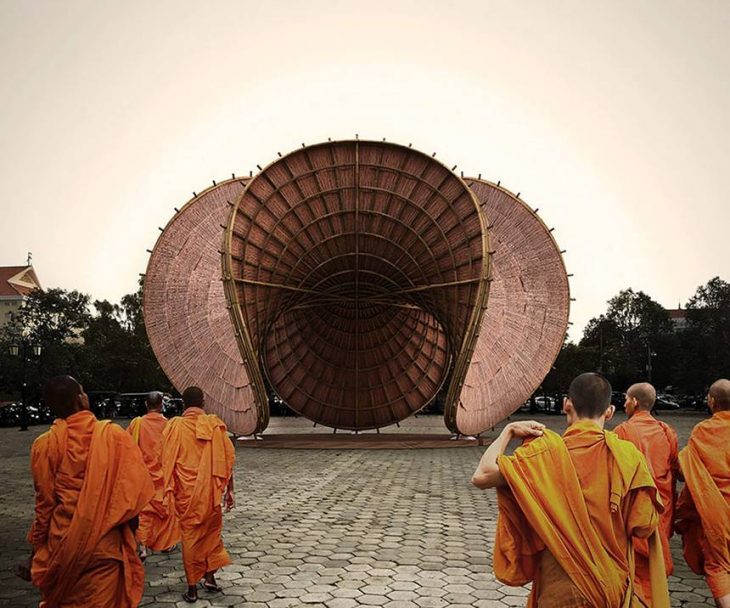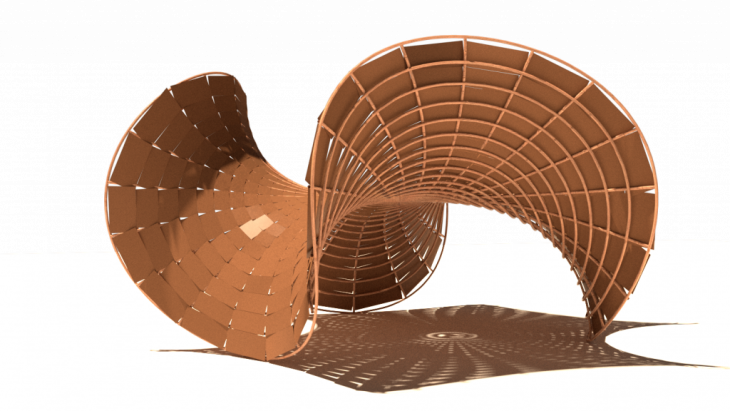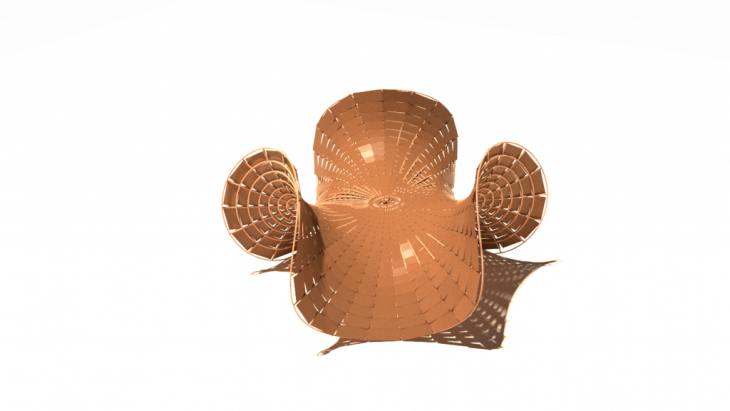“The form takes on the mathematical enneper surface, with a composite rim joist of individual bamboo planks continually bends forming the outer shell” (Hudson,2017)Images: (Poian, 2015)
PSEUDO CODE FOR THE CAMBOO PAVILLION :
1. Choose “Enneper Surface” from LunchBox plug in to create the base surface
2. Connect a 3 value slider to the R parameter to define the pattern for the eneper surface required.
3.  For the Domain 1 use a Construct Domain tool to define the construction process of the parametric surface. Attach sliders from 0 to 1 to both start and end domains, (1 being the complete form)
4. For the Domain 2 use a Construct Domain tool with both start and end inputs using a Pi component, on which the domain start uses the expression -x (-Pi =2pi)
5. Multiply the Pi number with a 1 slider to obtain the complete eneper surface desired.
6. Use a “Scale NU” to scale the surface in the XY plane and in order to control the height, width and lenght.
7. Create a bounding “Box” for the surface.
8. Use “Deconstruct Brep” to take the edges of the surface using a “List Item” connected to the “Edges” output.
9. The “List Item” component will provide us with the height, width and lenght of the surface.
10. Connect a “Curve” and  “Number” to the “List Item” output to know the witdh dimension. The dimension is provided in the “Number” component.
11. Add a “Division” component that inputs the “Number” and a number slider with the desired dimension. Output the “Division” to the “Scale X” input of the “Deconstruct Brep” component.
12. Copy and Paste this process to repeat the logic for both lenght and height.
13. Connect each retion to the other “Scale Y” and “Scale X” Inputs of the “Deconstruct Brep” component.
14. Creat a “Surface” to define the base surface of the pavillion.
15. Create an “Iso Curve” to the surface which will define the U curves and V curves on the surface in the same direction. This curves will draw the pipes on the Camboo Pavillion
16. Reparamaterize the “Surface” so the domain is from 0 to 1.
17. Use the “Construct Point” component to start the management of both U and V curves.
18. Use a “Range” component to define the amount and distribution of the curves, through a “Slider” and a “Graph Mapper”. This will define the U curve (X coordinate)
19. Create a “Pipe” connected to the V Isocurve output of the “Iso Curve” component.
20. Use a number slider for the radius of the pipe which will define the thickness.
21. The second pipe sits on the first pipe, therefore it is necessary to create a second surface that sits on the first one. For this “Offset” this first surface.
22. Use a “Multiplication” component to multiply by 2 the slider previously used to define the thickness of the “Pipe”
23. Set this “Multiplication” to the Distance input of “Offset” component. This will define the distance between both pipes.
24.Use the Expression -x to move the surface outwards.
25.Repeat the same tecnique for the ISO Curve to the second pipe copying and pasting onto the offset surface. Reparameterize this surface.
26. Change the Graph Mapper here to the Y coordinate (V curve) on the “Construct Point” component.
27. Increase the Steps for the “Range” slider to obtain the desire pipes for the Pavillion.
28. Copy the “Pipe” component and connect it to the U Curve on the “Iso Curve” component.
29. To empty the center of the pavillion create a “Sub-Curve” component to minimize the lenght of the pipes.
30. Bake each pipe into separate layers.
31. To create the realistic surface of the Camboo Pavillion find the offset surface and create an “Offset” component to this surface in order to create the outer layer.
32. Use the same distance input as as the previous offset.
33.Give a -x expression to this offset to create the surface outwards.
34. Add a “Quad Panels” component to create the panels of the surface.
35. Define the U and V divisions inputs with sliders according with the existing structural pipes.
36. Bake this final surface
37. Animate both Pipes and Quad Surface.References:
Poian, L. (2015). Camboo Pavillion. [image] Available at:

[Accessed 5 Dec. 2018].